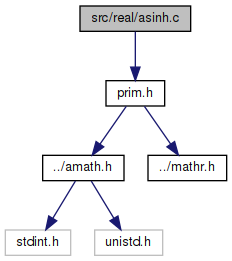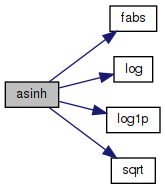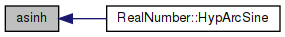amath  1.8.5 Simple command line calculator
asinh.c File Reference

Inverse hyperbolic sine function. More...

`#include "prim.h"`
Include dependency graph for asinh.c:Go to the source code of this file.

## Functions

double asinh (double x)
Inverse hyperbolic sine function. More...

## Variables

static const double one = 1.00000000000000000000e+00

static const double ln2 = 6.93147180559945286227e-01

static const double huge = 1.00000000000000000000e+300

## Detailed Description

Inverse hyperbolic sine function.

Definition in file asinh.c.

## ◆ asinh()

 double asinh ( double x )

Inverse hyperbolic sine function.

```Method
Based on
asinh(x) = sign(x) * log [ |x| + sqrt(x*x+1) ]```
```    we have
asinh(x) = x  if  1+x*x=1,
= sign(x)*(log(x)+ln2)) for large |x|, else
= sign(x)*log(2|x|+1/(|x|+sqrt(x*x+1))) if|x|>2, else
= sign(x)*log1p(|x| + x^2/(1 + sqrt(1+x^2)))
```

Definition at line 68 of file asinh.c.

References fabs(), huge, ln2, log(), log1p(), one, and sqrt().

Referenced by RealNumber::HypArcSine().

69 {
70  double t, w;
71  int32_t hx, ix;
72  GET_HIGH_WORD(hx, x);
73  ix = hx & 0x7fffffff;
74  if (ix >= 0x7ff00000)
75  return x + x; /* x is inf or NaN */
76  if (ix < 0x3e300000)
77  { /* |x|<2**-28 */
78  if (huge + x > one)
79  return x; /* return x inexact except 0 */
80  }
81  if (ix > 0x41b00000)
82  { /* |x| > 2**28 */
83  w = log(fabs(x)) + ln2;
84  }
85  else if (ix > 0x40000000)
86  { /* 2**28 > |x| > 2.0 */
87  t = fabs(x);
88  w = log(2.0 * t + one / (sqrt(x * x + one) + t));
89  }
90  else
91  { /* 2.0 > |x| > 2**-28 */
92  t = x * x;
93  w = log1p(fabs(x) + t / (one + sqrt(one + t)));
94  }
95  if (hx > 0)
96  return w;
97  else
98  return -w;
99 }
#define GET_HIGH_WORD(i, d)
Get the more significant 32 bit int from a double.
Definition: prim.h:167
static const double ln2
Definition: asinh.c:50
static const double huge
Definition: asinh.c:51
static const double one
Definition: asinh.c:49
double log1p(double x)
Definition: log1p.c:122
double sqrt(double x)
Square root function.
Definition: sqrt.c:119
double fabs(double x)
Returns the absolute value of x.
Definition: fabs.c:51
double log(double x)
Natural logarithm function (base e)
Definition: log.c:109
Here is the call graph for this function:Here is the caller graph for this function:## ◆ huge

 const double huge = 1.00000000000000000000e+300
static

Definition at line 51 of file asinh.c.

Referenced by asinh().

## ◆ ln2

 const double ln2 = 6.93147180559945286227e-01
static

Definition at line 50 of file asinh.c.

Referenced by asinh().

## ◆ one

 const double one = 1.00000000000000000000e+00
static

Definition at line 49 of file asinh.c.

Referenced by asinh().